Next: Chandrasekhar Limit Up: Quantum Statistics Previous: Sommerfeld Expansion

# White-Dwarf Stars

A main-sequence hydrogen-burning star, such as the Sun, is maintained in equilibrium via the balance of the gravitational attraction tending to make it collapse, and the thermal pressure tending to make it expand. Of course, the thermal energy of the star is generated by nuclear reactions occurring deep inside its core. Eventually, however, the star will run out of burnable fuel, and will, therefore, start to collapse, as it radiates away its remaining thermal energy. What is the ultimate fate of such a star?

A burnt-out star is basically a gas of electrons and ions. As the star collapses, its density increases, so the mean separation between its constituent particles decreases. Eventually, the mean separation becomes of order the de Broglie wavelength of the electrons, and the electron gas becomes degenerate. Note, that the de Broglie wavelength of the ions is much smaller than that of the electrons (because of the ions' much larger mass), so the ion gas remains non-degenerate. Now, even at zero temperature, a degenerate electron gas exerts a substantial pressure, because the Pauli exclusion principle prevents the mean electron separation from becoming significantly smaller than the typical de Broglie wavelength. (See Section 8.15 and Exercise 18.) Thus, it is possible for a burnt-out star to maintain itself against complete collapse under gravity via the degeneracy pressure of its constituent electrons. Such stars are termed white-dwarfs. Let us investigate the physics of white-dwarfs in more detail.

The total energy of a white-dwarf star can be written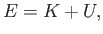(8.192)

where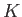is the total kinetic energy of the degenerate electrons (the kinetic energy of the ions is negligible), and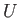is the gravitational potential energy. Let us assume, for the sake of simplicity, that the density of the star is uniform. In this case, the gravitational potential energy takes the form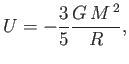(8.193)

where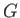is the gravitational constant,the stellar mass, and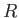the stellar radius.

Let us assume that the electron gas is highly degenerate, which is equivalent to taking the limit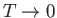. In this case, we know, from Section 8.15, that the Fermi momentum can be written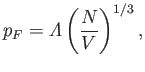(8.194)

where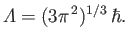(8.195)

Here,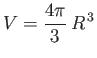(8.196)

is the stellar volume, and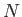the total number of electrons contained in the star. Furthermore, the number of electron states contained in an annular radius of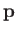-space lying between radiiand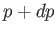is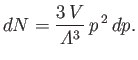(8.197)

(See Exercise 20.) Hence, the total kinetic energy of the electron gas can be written(8.198)

where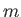is the electron mass. It follows that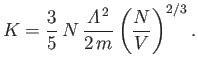(8.199)

The interior of a white-dwarf star is composed of atoms like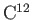and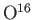that contain equal numbers of protons, neutrons, and electrons. Thus,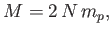(8.200)

where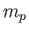is the proton mass.

Equations (8.192), (8.193), (8.195), (8.196), (8.199), and (8.200) can be combined to give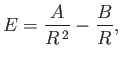(8.201)

where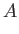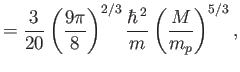(8.202)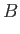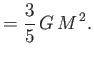(8.203)

The equilibrium radius of the star,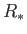, is that which minimizes the total energy,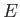. In fact, it is easily demonstrated that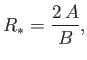(8.204)

which yields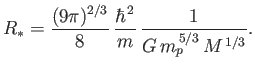(8.205)

The previous formula can also be written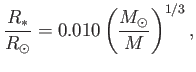(8.206)

where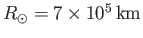is the solar radius, and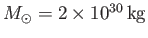the solar mass. It follows that the radius of a typical solar-mass white-dwarf is about 7000km: that is, about the same as the radius of the Earth. The first white-dwarf to be discovered (in 1862) was the companion of Sirius. Nowadays, thousands of white-dwarfs have been observed, all with properties similar to those described previously.Next: Chandrasekhar Limit Up: Quantum Statistics Previous: Sommerfeld Expansion
Richard Fitzpatrick 2016-01-25